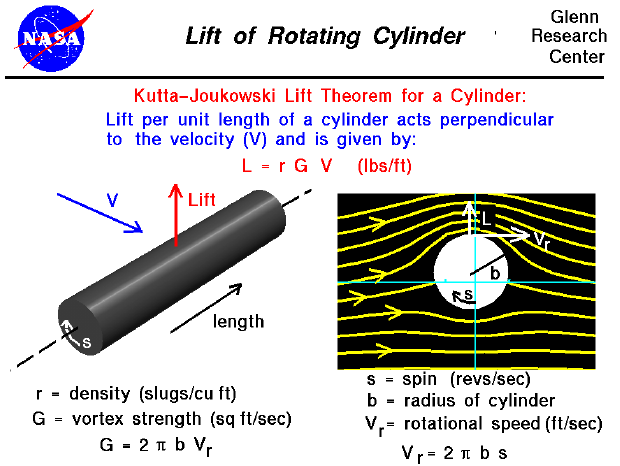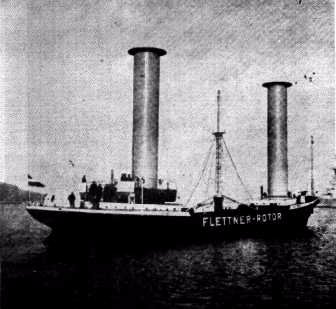All that is necessary to create lift is to turn a flow of air. We are familiar with the lift generated by an airplane wing or a curving baseball. But a simple rotating cylinder will also create lift. In fact, because the flow field associated with a rotating cylinder is two dimensional, it is much easier to understand the basic physics of this problem than the more complex three dimensional aspects of a curveball.

However, the details of how a rotating cylinder creates lift are still pretty complex. Next to any surface, the molecules of the air will stick to the surface as discussed in the properties of air slide. This thin layer of molecules will entrain or pull the surrounding flow in the direction that the surface moves. If we put a cylinder that is rotating about the longitudinal axis (a line perpendicular to the circular cross section) into a fluid, it would eventually create a spinning, vortex-like flow around the cylinder. If we then set the fluid in motion, the uniform velocity flow field can be added to the vortex flow. The right part of the slide shows a view of the flow as if we were moving with the cylinder looking down the longitudinal axis. The cylinder appears stationary and the flow moves from left to right. The cylinder rotates clockwise. So the free stream flow over the top of the cylinder is assisted by the induced flow; the free stream flow below the cylinder is opposed by the induced flow. The streamlines around the cylinder are distorted because of the spinning. If the cylinder were not spinning, the streamlines would be symmetric top and bottom. The net turning of the flow has produced an upward force. Because of the change to the velocity field, the pressure field will also be altered around the cylinder. The magnitude of the force can be computed by integrating the surface pressure times the area around the cylinder. The direction of the force is perpendicular to the flow direction.

The magnitude of the force was determined by two early aerodynamicists, Kutta in Germany and Joukowski in Russia. The lift equation for a rotating cylinder bears their names. The equation states that the lift (L) per unit length along the cylinder is directly proportional to the velocity (V) of the flow, the density (r) of the flow, and the strength of the vortex (G) that is established by the rotation.

L = r * V * G

The equation gives lift-per-unit length because the flow is two dimensional. (Obviously, the longer the cylinder the greater the lift.) Determining the vortex strength (G) takes a little more math. The vortex strength equals the rotational speed (Vr) times the circumference of the cylinder. If b is the radius of the cylinder,

G = 2.0 * pi * b * Vr

where pi =3.14159. The rotational speed (Vr) is equal to the circumference of the cylinder times the spin (s) of the cylinder.

Vr = 2.0 * pi * b * s

Let's investigate the lift of a rotating cylinder by using a Java simulator.

The left window shows an edge view of a cylinder placed in a flow of air. The cylinder is two feet in diameter and 20 feet long and the air is flowing past this cylinder at 100 miles per hour. You can rotate the cylinder by using the slider below the view window or by backspacing over the input box, typing in your new value and hitting the Enter key on the keyboard. On the right is a graph of the lift versus spin. The red dot shows your conditions. Below the graph is the numerical value of the lift. You can display either the lift value (in English or Metric units) or the lift coefficient by using the choice buttons surrounding the output box. Click on the choice button and select from the drop-menu.

As an experiment, set the spin to 100 rpm (revolutions per minute) and note the amount of lift. Now increase the spin to 200 rpm. Did the lift increase or decrease? Set the spin to -100 rpm. What is the value of lift? Which way would this cylinder move?

You can further investigate the lift of a cylinder, and a variety of other shapes by using the FoilSim II Java Applet. You can also download your own copy of FoilSim to play with for free.

WARNING: Be particularly aware of the simplifying assumptions that have gone into this analysis. This type of flow field is called an ideal flow field. It is produced by superimposing the flow field from an ideal vortex centered in the cylinder with a uniform free stream flow. There is no viscosity in this model (no boundary layer on the cylinder) even though this is the real origin of the circulating flow! In reality, the flow around a rotating cylinder is very complex. Depending on the ratio of rotational speed, free stream speed, viscosity of the fluid, and size of the cylinder, the flow off the rear of the cylinder can separate and become unsteady. BUT, the simplified model does give the first order effects; it gives an initial good prediction of the force on the cylinder when compared to experiments.

HISTORICAL NOTE: In the early 1920's the force from a rotating cylinder was used to power a sailing ship. The idea, proposed by Anton Flettner of Germany, was to replace the mast and cloth sails with a large cylinder rotated by an engine below deck. The idea worked, but the propulsion force generated was less than the motor would have generated if it had been connected to a standard marine propeller! Here's a picture of the ship provided by Brian Adkins, BAE, Georgia Tech, 1993.Jaques Cousteau and the Cousteau Society proposed a similar ship, named Calypso II, to explore this and other oceanographic technologies in the 1990's.Guided Tours